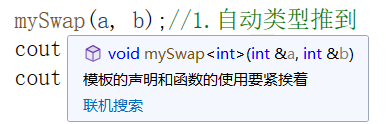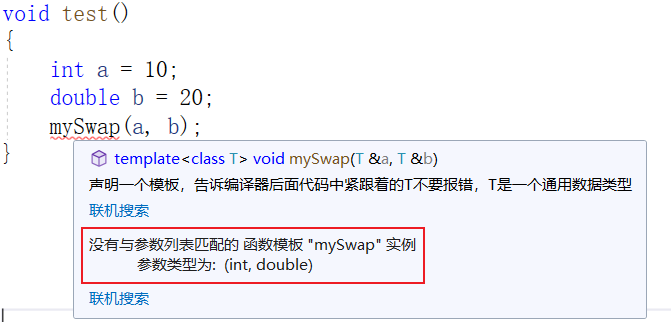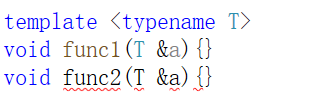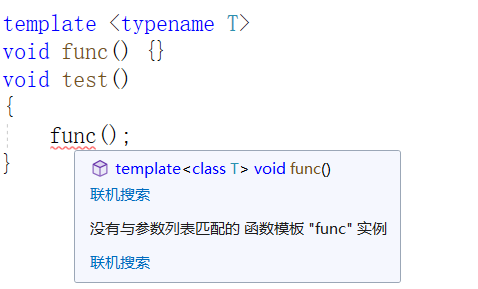# C++ 函数模板### •共勉

“那些疯狂到以为自己能够改变世界的人，才能真正改变世界。”

### •函数模板

#### 介绍

• C++另一种编程思想称为 泛型编程 ，主要利用的技术就是模板
• C++提供两种模板机制：函数模板 和 类模板

#### 函数模板

```template <typename T>//声明一个模板，告诉编译器后面代码中紧跟着的 T 不要报错，T 是一个通用数据类型

• template：声明创建模板
• typename：表面其后面的符号是一种数据类型
• T：通用的数据类型，名称可以替换，通常为大写字母

建立一个通用函数，其函数返回值类型和形参类型可以不具体制定，用一个虚拟的类型来代表。

例如，对于如下交换函数：

```void swapInt(int &a, int &b)
{
int tmp = a;
a = b;
b = tmp;
}```

我们传递的参数只能是整数类型，如果我们想要交换 double 类型的呢？

那当然是重写一份啦~

```void swapDouble(double& a, double& b)
{
double tmp = a;
a = b;
b = tmp;
}```

对于这两份代码，你会发现除了参数类型不同外，交换的步骤都是相同的。

那如果要交换 short 类型的呢，在重写一份吗？

会不会过于啰嗦，下面就引出函数模板的强大之处。

我们可以这么写：

```template <typename T>//声明一个模板，告诉编译器后面代码中紧跟着的T不要报错，T是一个通用数据类型
void mySwap(T& a, T& b)
{
T tmp = a;
a = b;
b = tmp;
}```

利用函数模板实现数据交换，有两种调用方式：

• 自动类型推导
• 显示指定类型
```void test()
{
int a = 10;
int b = 20;

mySwap(a, b);//1.自动类型推到
cout << "a = " << a << endl;
cout << "b = " << b << endl;

double c = 30;
double d = 40;

mySwap<double>(c, d);//2.显示指定类型
cout << "c = " << c << endl;
cout << "d = " << d << endl;
}```

对于方式 1，编译器会自动识别传递的参数类型，并做相应的调整：传递的参数类型必须是同种类型的，即必须推导出一致的数据类型 T 才可以使用。

比如就不能通过  mySwap  函数交换 int 和 double 类型的数据：

```void test()
{
int a = 10;
double b = 20;
myswap(a, b);
}```

由于 a,b 不是同种类型的，在编译阶段就会报错：提示推导不出一致的 T 类型。

必须遵循模板的声明和函数的声明一对一使用，即模板的声明只作用于紧挨着的函数声明。

比如下面这种代码就会报错：

```template <typename T>
void func1(T &a){}
void func2(T &a){}```因为声明的函数模板 T 只对  func1  起作用，如果  func2  也想用，必须重新声明：

```template <typename T>
void func1(T &a) {}

template <typename T>
void func2(T &a) {}```

注意事项 3

模板必须要确定出 T 的数据类型，才可以使用。

比如如下代码就会报错：

```template <typename T>
void func() {}
void test()
{
func();
}```

因为函数  func()  的声明紧挨着模板的声明，所以他俩就是一对一的关系，那么要想调用  func ，就必须给出 T 的数据类型；

不然编译阶段都不通过。对于这种不含参数的函数模板，必须使用 显示指定类型 来调用：

```template <typename T>
void func() {}
void test()
{
func<int>();
}```

其中 <int> 随便写个数据类型就行，作用就是告诉函数模板传递的 T 为  XX 类型 。

### •学以致用

有了函数模板的知识储备，下面来写一个排序函数，不管是传入  int 类型  还是  char 类型  都可以实现升序排列。

#### 选择排序

```template <typename T>
void mySwap(T& a, T& b)
{
T tmp = a;
a = b;
b = tmp;
}
template <typename T>
void mySort(T arr[],int first,int last)//order [first, last)
{
for (int i = first; i < last; i++)//选择排序
{
int minIndex = i;
for (int j = i + 1; j < last; j++)
{
if (arr[j] < arr[minIndex])
minIndex = j;
}
mySwap(arr[i], arr[minIndex]);
}
}```

#### 调用该排序函数

```template <typename T>
void print(const T arr[], int first, int last)//输出数组[first, last)
{
for (int i = first; i < last; i++)
cout << arr[i] << " ";
cout << endl;
}
void test1()
{
//测试对整形数组进行排序
int arr;
for (int i = 0; i < 10; i++)
arr[i] = rand()%20;//随机产生[0~20)之间的随机数

cout << "排序前：";
print(arr, 0, 10);
mySort(arr, 0, 10);
cout << "排序后：";
print(arr, 0, 10);
}
void test2()
{
//测试对字符型数组进行排序
char arr;
for (int i = 0; i < 10; i++)
arr[i] = 'A'+rand() % 20;//随机产生[0~20)之间的随机数

cout << "排序前：";
print(arr, 0, 10);
mySort(arr, 0, 10);
cout << "排序后：";
print(arr, 0, 10);
}```

#### CODE

```#include<bits/stdc++.h>
using namespace std;

template <typename T>
void mySwap(T& a, T& b)
{
T tmp = a;
a = b;
b = tmp;
}
template <typename T>
void mySort(T arr[],int first,int last)//order [first, last)
{
for (int i = first; i < last; i++)//选择排序
{
int minIndex = i;
for (int j = i + 1; j < last; j++)
{
if (arr[j] < arr[minIndex])
minIndex = j;
}
mySwap(arr[i], arr[minIndex]);
}
}
template <typename T>
void print(const T arr[], int first, int last)//输出数组[first, last)
{
for (int i = first; i < last; i++)
cout << arr[i] << " ";
cout << endl;
}
void test1()
{
//测试对整形数组进行排序
int arr;
for (int i = 0; i < 10; i++)
arr[i] = rand()%20;//随机产生[0~20)之间的随机数

cout << "排序前：";
print(arr, 0, 10);
mySort(arr, 0, 10);
cout << "排序后：";
print(arr, 0, 10);
}
void test2()
{
//测试对字符型数组进行排序
char arr;
for (int i = 0; i < 10; i++)
arr[i] = 'A'+rand() % 20;//随机产生[0~20)之间的随机数

cout << "排序前：";
print(arr, 0, 10);
mySort(arr, 0, 10);
cout << "排序后：";
print(arr, 0, 10);
}
int main()
{
test1();
test2();
return 0;
}```

### •结尾

咳咳，本次内容到此结束，完结撒花

2 total views,  1 views today